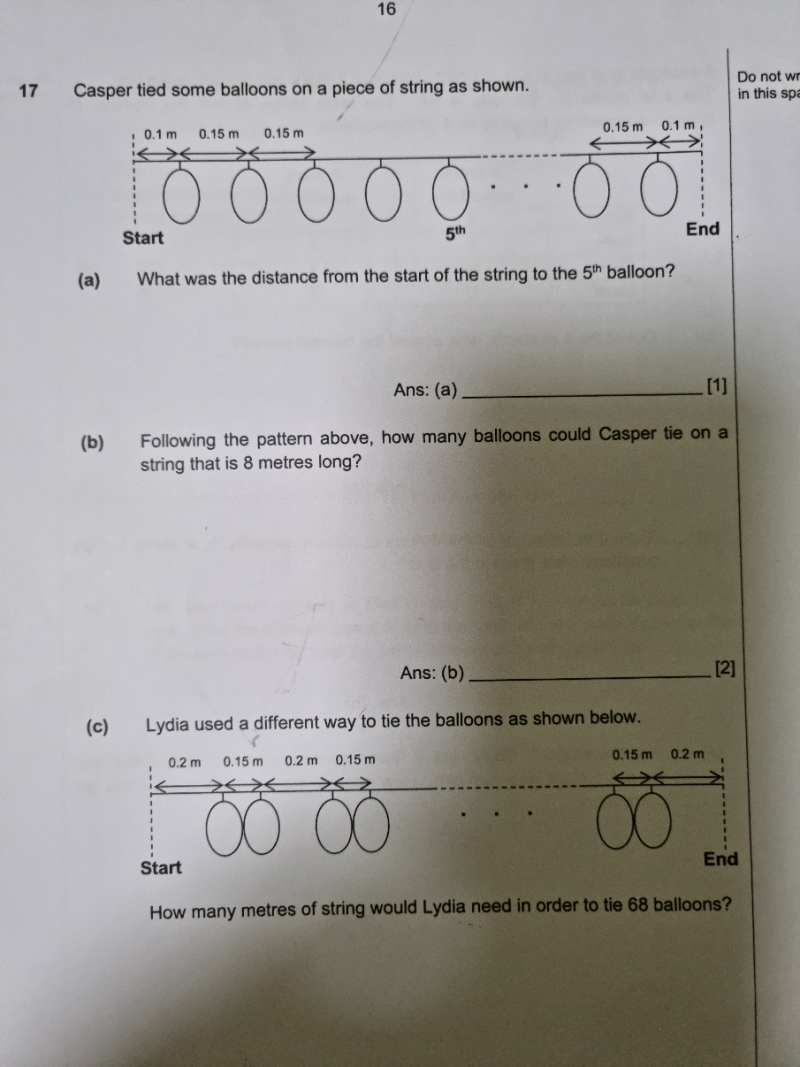# QuestionThank you.

(a)  Distance from start to 5th balloon = 0.1 + (0.15 x 4 ) = 0.1 + 0.6 = 0.7m

(b) 8.0 – 0.2 = 7.8   (0.1m on each end)

7.8/0.15 = 52 spaces

Hence number of balloons = 53

(c)  Space between 2 balloons = 0.15, Space between a pair of balloons = 0.2

For 68 balloons,

Space between 2 balloons =  68/2 x 0.15 = 5.1

Space between a pair of balloons = 33 x 0.2 = 6.6

Start and end space = 0.2 x 2 = 0.4

Total length of strength required = 5.1 + 6.6 + 0.4 = 12.1m